# Area of a Triangle (1/2abSinC) Worksheet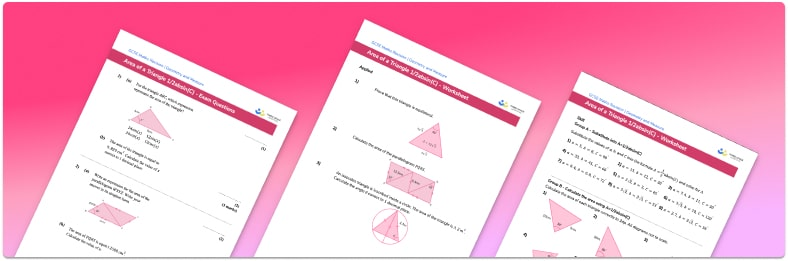• This field is for validation purposes and should be left unchanged.

You can unsubscribe at any time (each email we send will contain an easy way to unsubscribe). To find out more about how we use your data, see our privacy policy.

Help your students prepare for their Maths GCSE with this free area of a triangle using sine worksheet of 30 questions and answers

• Section 1 of the area of a triangle using sine worksheet contains 27 skills-based questions, in 3 groups to support differentiation
• Section 2 contains 3 applied area of a triangle using sine questions with a mix of word problems and deeper problem solving questions
• Answers and a mark scheme for all questions are provided
• Questions follow variation theory with plenty of opportunities for students to work independently at their own level
• All questions created by fully qualified expert secondary maths teachers
• Suitable for GCSE maths revision for AQA, OCR and Edexcel exam boards
• Math worksheets on related topics such as metric units, polygons, types of triangles, area of trapezoids and area of compound shapes are also available

### Area of a triangle using sine at a glance

The formula Area = (1/2)absin(C) can be used to find the area of any triangle. This is useful when we don’t know the length of the base or the height of the triangle. When using this formula, the sides of the triangle are labelled a, b and c and the angle opposite side c is labelled C.

The formula can be used to find the area of equilateral triangles, isosceles triangles and scalene triangles. We usually would not use it to find the area of a right triangle since we can easily identify the height and base of the triangle when there is a right angle.

To find the area of the triangle, we substitute the side lengths a and b and the angle C into the formula and perform the calculation. It is likely that the answer will be a decimal so be sure to check how many decimal places or significant figures are required for the answer.

Looking forward, students can then progress to additional geometry worksheets, for example, a 3D shapes worksheet or .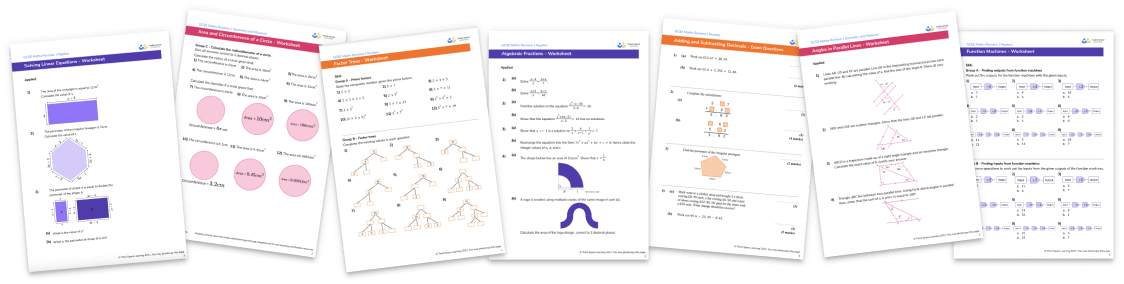For more teaching and learning support on Geometry our GCSE maths lessons provide step by step support for all GCSE maths concepts.

## Related worksheets

Exact Trig Values Worksheet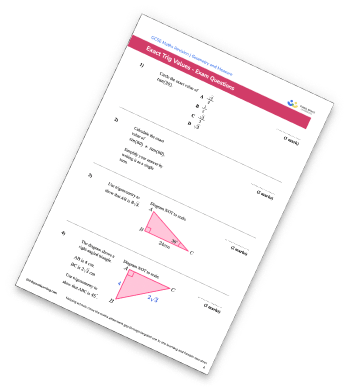Hypotenuse Worksheet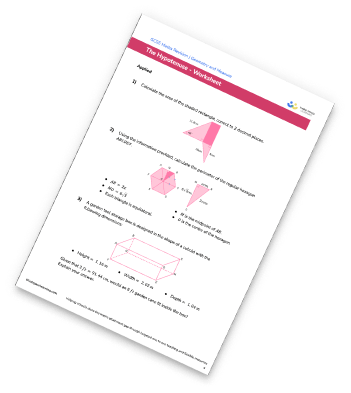Trigonometry Worksheets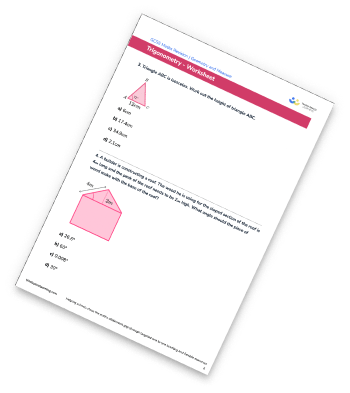Sine Rule Worksheet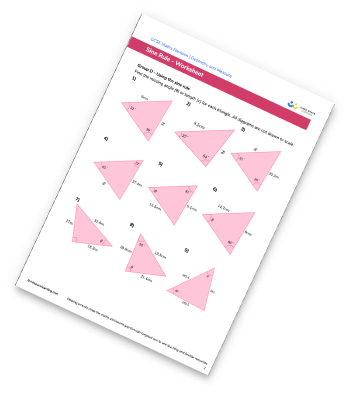## Do you have KS4 students who need more focused attention to succeed at GCSE?There will be students in your class who require individual attention to help them succeed in their maths GCSEs. In a class of 30, it’s not always easy to provide.

Help your students feel confident with exam-style questions and the strategies they’ll need to answer them correctly with our dedicated GCSE maths revision programme.

Lessons are selected to provide support where each student needs it most, and specially-trained GCSE maths tutors adapt the pitch and pace of each lesson. This ensures a personalised revision programme that raises grades and boosts confidence.

Find out more